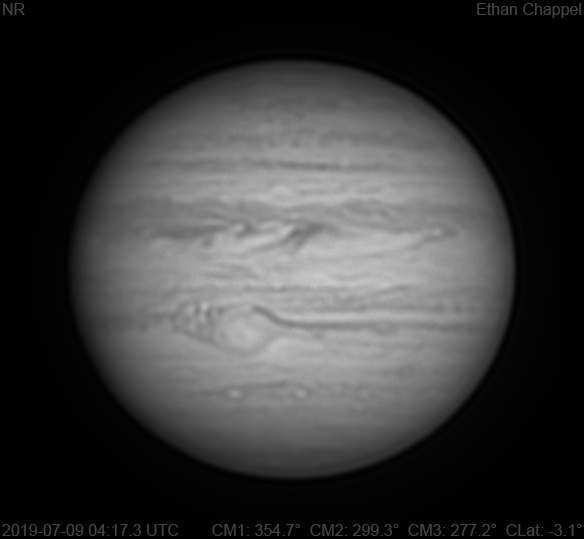# Jupiter 2019-07-09 04:17 UTC

CM1: 354.70°

CM2: 299.30°

CM3: 277.20°

CLat: -3.10°

Description

The third night of excellent planetary imaging, but also the third night of breezy conditions too intense for the CGEM DX and C14, so this is another session using the C8.

Notes:

• White Oval Z is at the central meridian.
• The Great Red Spot has a small dark spot on its western edge.
• Two SEB rings are approaching the GRS from the east, carrying with them the potential for more flakes.
• The STB contains a small white oval with a dark boundary.

Equipment

ZWO ASI290MM

Celestron EdgeHD 8

Logs
```FireCapture v2.6  Settings
------------------------------------
Observer=Ethan Chappel
Camera=ZWO ASI290MM
Filter=IR
Profile=Jupiter
Diameter=44.86"
Magnitude=-2.54
CMI=355.5° CMII=300.1° CMIII=277.9°  (during mid of capture)
FocalLength=3700mm (F/13)
Resolution=0.16"
Filename=2019-07-09-0418_4-EC-IR-Jup.ser
Date=2019_07_09
Start=04_17_26.992
Mid=04_18_26.996
End=04_19_27.000
Start(UT)=04_17_26.992
Mid(UT)=04_18_26.996
End(UT)=04_19_27.000
Duration=120.008s
Date_format=yyyy_MM_dd
Time_format=HH_mm_ss
LT=UT -6h
Frames captured=7997
File type=SER
Binning=no
Bit depth=8bit
Debayer=no
ROI=428x362
ROI(Offset)=0x0
FPS (avg.)=66
Shutter=15.00ms
Gain=290 (48%)
Gamma=50
SoftwareGain=10 (off)
AutoExposure=off
Brightness=1 (off)
USBTraffic=40 (off)
AutoHisto=75 (off)
HighSpeed=off
FPS=100 (off)
AutoGain=off
Histogramm(min)=0
Histogramm(max)=133
Histogramm=52%
Noise(avg.deviation)=0.79
AutoAlign=false
PreFilter=none
Limit=120 Seconds
Sensor temperature=36.3°C
FireCapture v2.6  Settings
------------------------------------
Observer=Ethan Chappel
Camera=ZWO ASI290MM
Filter=IR
Profile=Jupiter
Diameter=44.86"
Magnitude=-2.54
CMI=354.2° CMII=298.7° CMIII=276.6°  (during mid of capture)
FocalLength=3750mm (F/13)
Resolution=0.16"
Filename=2019-07-09-0416_2-EC-IR-Jup.ser
Date=2019_07_09
Start=04_15_15.095
Mid=04_16_15.099
End=04_17_15.104
Start(UT)=04_15_15.095
Mid(UT)=04_16_15.099
End(UT)=04_17_15.104
Duration=120.009s
Date_format=yyyy_MM_dd
Time_format=HH_mm_ss
LT=UT -6h
Frames captured=7998
File type=SER
Binning=no
Bit depth=8bit
Debayer=no
ROI=428x362
ROI(Offset)=0x0
FPS (avg.)=66
Shutter=15.00ms
Gain=290 (48%)
Gamma=50
SoftwareGain=10 (off)
AutoExposure=off
Brightness=1 (off)
USBTraffic=40 (off)
AutoHisto=75 (off)
HighSpeed=off
FPS=100 (off)
AutoGain=off
Histogramm(min)=0
Histogramm(max)=134
Histogramm=52%
Noise(avg.deviation)=0.79
AutoAlign=false
PreFilter=none
Limit=120 Seconds
Sensor temperature=36.3°C
```